Univerza na Primorskem Fakulteta za matematiko, naravoslovje in informacijske tehnologije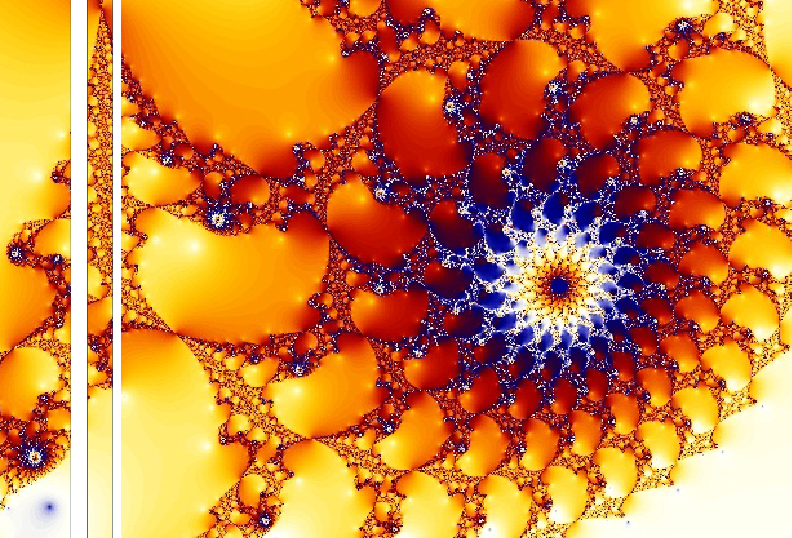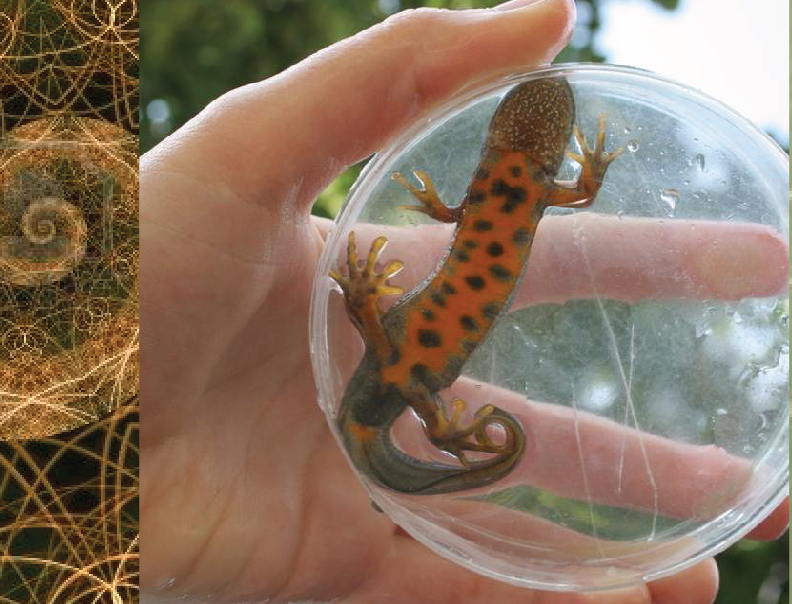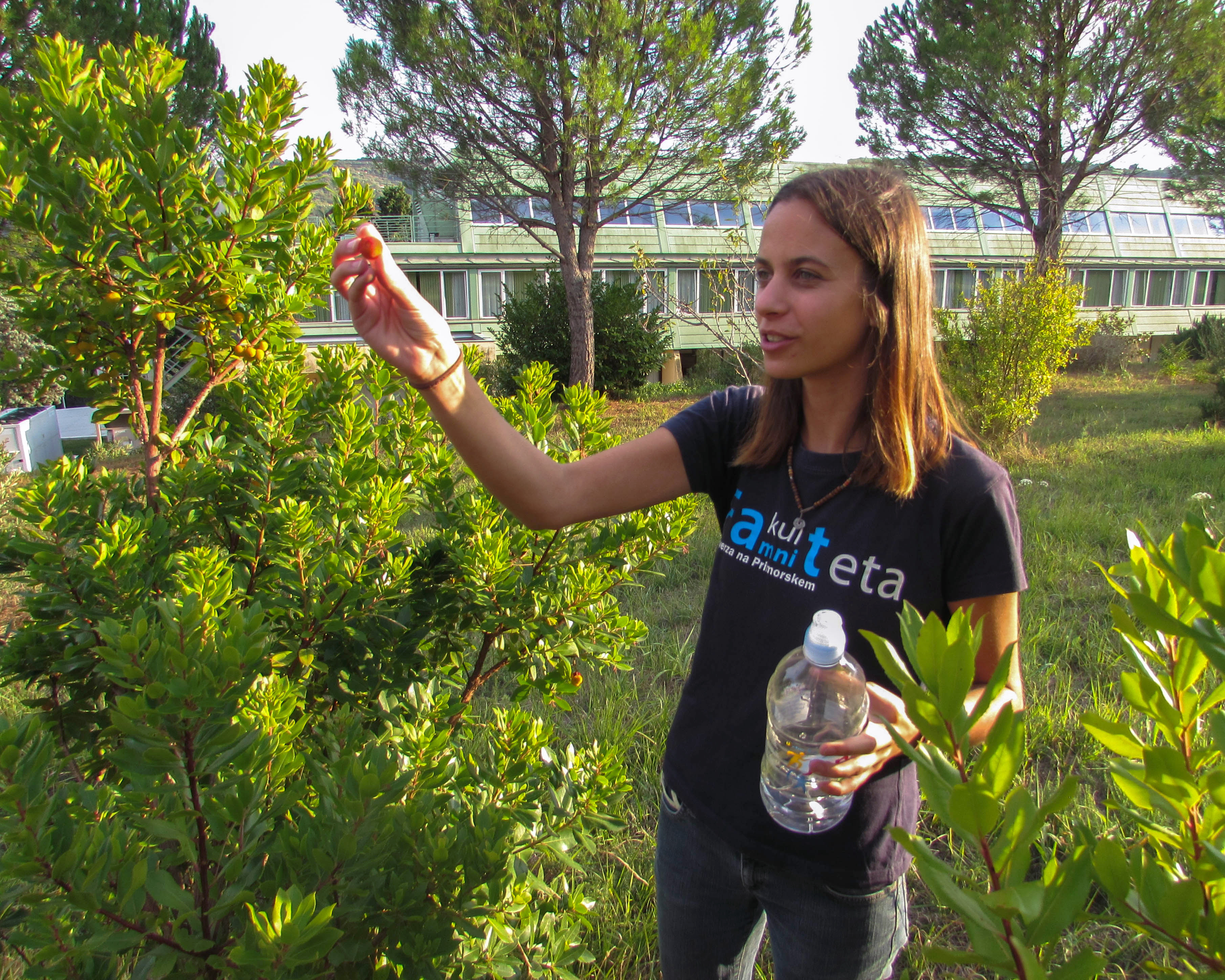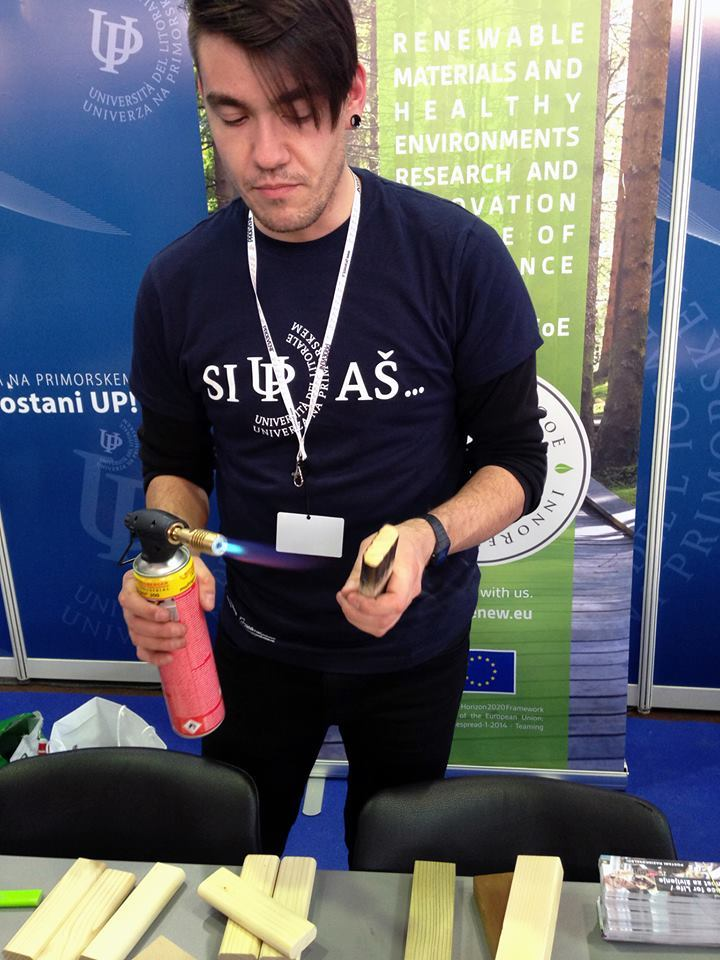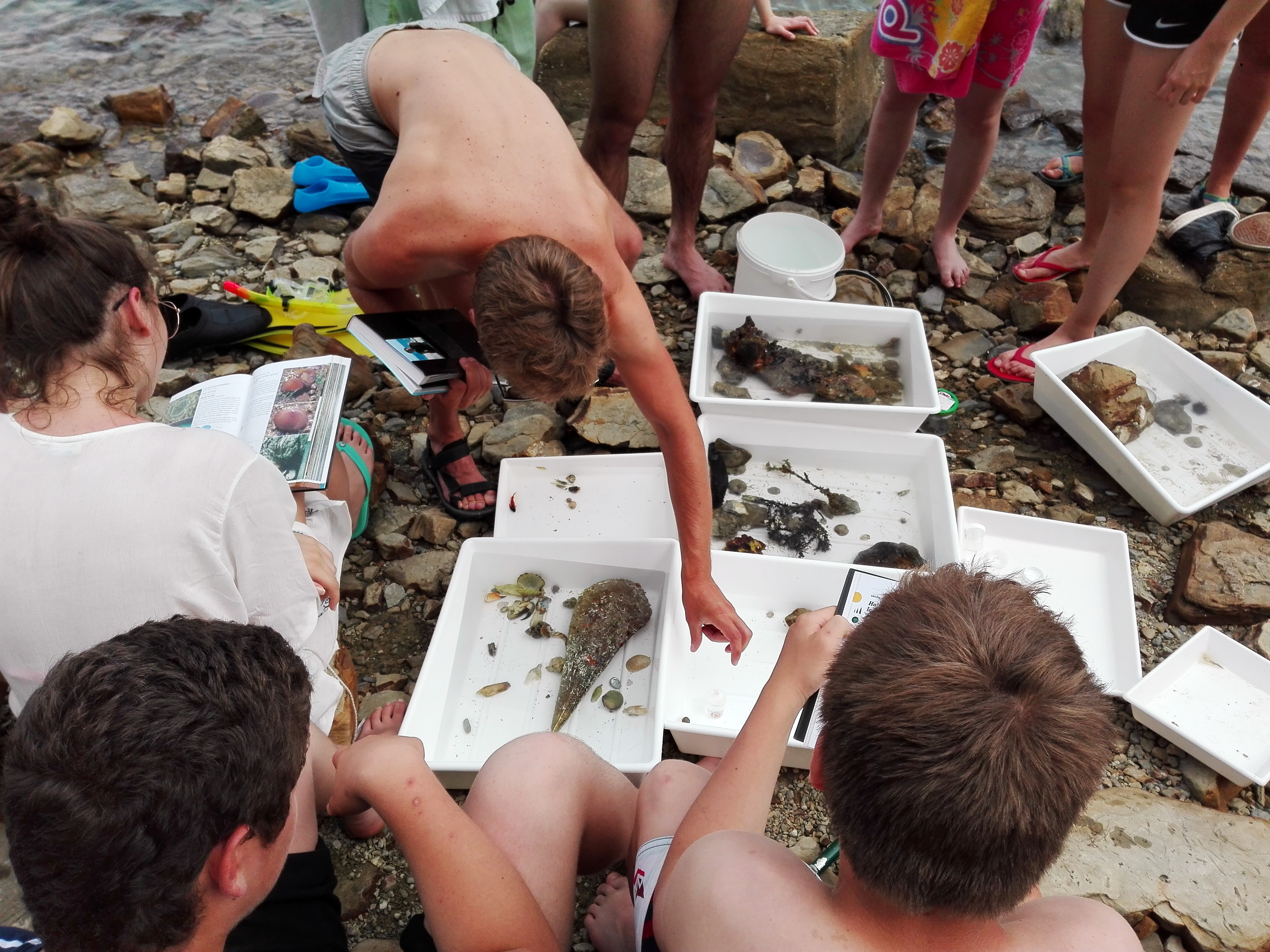SI | EN# Raziskovalni matematični seminar - Arhiv

 2021 2020 2019 2018 2017 2016 2015 2014 2013 2012 2011 2010
 1 2 3 4 5 6 7 8 9 10 11 12
Datum in ura / Date and time: 20.12.12
(14:00-15:00)
Predavalnica / Location: Famnit-Semin (Seminar room)
Predavatelj / Lecturer: Prof. Andreas Brandstädt (University of Rostock, Germany)
Naslov / Title: On the complexity of some packing and covering problems in certain graph classes
Vsebina / Abstract:

Packing and covering problems in graphs and hypergraphs and their relationships belong to the most fundamental topics in combinatorics and graph algorithms and have a wide spectrum of applications in computer science, operations research and many other fields. Vertex Cover is a typical example of a covering problem, and Maximum Independent Set is a typical example of a packing problem, and the complexity of these two problems was investigated in thousands of papers.

Recently, there has been an increasing interest in problems combining packing and covering properties. For a hypergraph H = (V, E), Exact Cover is a classical problem asking for the existence of a subcollection E′ ⊆ E such that every vertex of V is contained in exactly one hyperedge from E′. It is well known that this problem is NP- complete even when the hyperedges contain only three vertices called Exact Cover by 3-Sets.

Among the problems combining packing and covering properties, there are the follo- wing variants of domination problems: Let G = (V, E) be a finite undirected and simple graph, and let N(G) denote the closed neighborhood hypergraph of G. A vertex set U ⊆ V is an efficient dominating set in G if the closed neighborhoods of vertices in U form an exact cover in N (G). An edge set M ⊆ E is an efficient edge dominating set in G if M is an efficient dominating set in the line graph L(G). Note that not every graph has an efficient dominating set (an efficient edge dominating set, respectively). The problem Efficient Domination (Efficient Edge Domination, respective- ly) asks for the existence of such a vertex set (edge set, respectively). It is well known that both problems are NP-complete, even for bipartite graphs. We give some new ca- ses where the problems are efficiently solvable by using structural properties of graph classes and using closure properties under the square operation, and we also generalize the problems to hypergraphs.

Datum in ura / Date and time: 11.12.12
(10:00-11:00)
Predavalnica / Location: FAMNIT-SEMIN
Predavatelj / Lecturer: prof. Pablo Spiga (University of Milano-Bicocca)
Naslov / Title: Is the theory of finite primitive groups finished?
Vsebina / Abstract:
 From time to time you hear people saying that the theory of finite primitive groups is dead (after theO'Nan-Scott theorem and the Classification of the Finite Simple Groups). In this talk, we present a result on cycle lengths that could have been discovered by Schur, or Wielandt or Manning. However, they didn't because such powerful results are not the end of the theory, but the beginning.

Datum in ura / Date and time: 3.12.12
(10:00)
Predavalnica / Location: FAMNIT-MP
Predavatelj / Lecturer: Dragan Marušič
Naslov / Title: Primitive groups of degree twice a prime number (part II)
Vsebina / Abstract:

We will give a short overview and discuss possible future directions with regards to the classification of strongly regular bicirculants, in relation to the problem of obtaining a CFSG-free proof of nonexistence of simply primitive groups of degree $2p$.

Datum in ura / Date and time: 26.11.12
(10:00)
Predavalnica / Location: FAMNIT-MP
Predavatelj / Lecturer: Dragan Marušič
Naslov / Title: Primitive groups of degree twice a prime number
Vsebina / Abstract:

We will give a short overview and discuss possible future directions with regards to the classification of strongly regular bicirculants,  in relation to the problem of obtaining a CFSG-free proof of nonexistence of simply primitive groups of degree $2p$.

Datum in ura / Date and time: 1.1.70
(Time: 10:00)
Predavalnica / Location: Location: FAMNIT-MP
Predavatelj / Lecturer: Lecturer: Dragan Marušič
Naslov / Title: Title: Primitive groups of degree twice a prime number
Vsebina / Abstract:

Abstract: We will give a short overview and discuss possible future directions with regards to the classification of strongly regular bicirculants, in relation to the problem of obtaining a CFSG-free proof of nonexistence of simply primitive groups of degree $2p$.

Datum in ura / Date and time: 19.11.12
(9:00)
Predavalnica / Location: Seminar room at Galeb (Kettejeva 1, Koper)
Predavatelj / Lecturer: Prof. Endre Boros (RUTCOR, Rutgers University, USA)
Naslov / Title: Quadratization of Pseudo-Boolean Functions
Vsebina / Abstract:

Set functions, i.e., real mappings form the family of subsets of a finite set to the reals are known and widely used in discrete mathematics for almost a century, and in particular in the last 50 years. If we replace a finite set with its characteristic vector, then the same set function can be interpreted as a mapping from the set of binary vectors to the reals. Such mappings are called pseudo-Boolean functions and were introduced in the works of Peter L. Hammer in the 1960s (Hammer and Rudeanu, 1968). Pseudo-Boolean functions are different from set functions, only in the sense that their algebraic representation, a multilinear polynomial expression, is usually assumed to be available as an input representation.

The problem of minimizing a pseudo-Boolean function (over the set of binary vectors) appears to be the common form of numerous optimization problems, including the well-known MAX-SAT and MAX-CUT problems, and have applications in areas ranging from physics through chip design to computer vision, etc.

Some of these applications lead to the minimization of a quadratic pseudo-Boolean function, and hence such quadratic binary optimization problems received ample attention in the past decades. One of the most frequently used technique is based on roof-duality (Hamer, Hansen, Simeone, 1984), and aims at finding in polynomial time a simpler form of the given quadratic minimization problem, by fixing some of the variables at their provably optimum value (persistency) and decomposing the residual problem into variable disjoint smaller subproblems (Boros and Hammer, 1989). The method in fact was found very effective in computer vision problems, where frequently it can fix up to 80-90% of the variables at their provably optimum value (Boros, Hammer, Sun and Tavares, 2008). This algorithm was used by computer vision experts and a very efficient implementation, called QPBO, is freely downloadable (Rother, Kolmogorov, Lempitsky and Szummer, 2007).

In many applications of pseudo-Boolean optimization, in particular in vision problems, the objective function is a higher degree multilinear polynomial. For such problems there are substantially fewer effective techniques available. In particular, there is no analogue to the persistencies (fixing variables at their provably optimum value) provided by roof-duality for the quadratic case. On the other hand, more and more applications would demand efficient methods for the minimization of such higher degree pseudo-Boolean functions. This increased interest, in particular in the computer vision community, lead to a systematic study of methods to reduce a higher degree minimization problem to a quadratic one. We report on the most recent techniques and the computational success of those.

Joint research with Alex Fix (Cornell University), Aritanan Gruber (Rutgers University), Gabriel Tavares (FICO, Xpress-MP), and Ramin Zabih (Cornell University).

Datum in ura / Date and time: 12.11.12
(10:00)
Predavalnica / Location: Seminar room at Galeb (Kettejeva 1, Koper)
Predavatelj / Lecturer: dr. Bojan Kuzma
Naslov / Title: On maps which do not increase the spectum of difference of matrices
Vsebina / Abstract:

We will show that maps, which do not increase the spectra of complex matrices (in a sense that $Sp(Phi(A)-Phi(B)) subseteq Sp(A-B)$) are automatically linear and bijective. Although the problem is elementary, its proof relies on deep results from the theory of analitic functions.

Datum in ura / Date and time: 22.10.12
(10:30-10:55)
Predavalnica / Location: Seminar room at Galeb (Kettejeva 1, Koper).
Predavatelj / Lecturer: Riste Škrekovski (FMF and IMFM, Ljubljana, Slovenia)
Naslov / Title: Some results on network centralitiy indicies
Vsebina / Abstract:

Centrality is used to quantify an intuitive feeling that in most networks some vertices are more central than others. In the talk we consider some graph theoretical results on closeness, betweenness centrality, and eccentricity.

Datum in ura / Date and time: 5.11.12
(10:00)
Predavalnica / Location: Seminar room at Galeb (Kettejeva 1, Koper)
Predavatelj / Lecturer: dr. Dragan Stevanović
Naslov / Title: Spectral approach to network partitioning problems
Vsebina / Abstract:

An important part of the network analysis are network partitioning  problems, which come in the forms of partitioning, finding maximum cut and detecting communities. There are well known, simple spectral heuristics for all three problems, which assign nodes to an appropriate part based on the sign of the eigenvector corresponding to an extremal eigenvalue of a particular network matrix. We will discuss a general framework in which these heuristics work and suggests a way for their further improvement.

All welcome!

Datum in ura / Date and time: 29.10.12
(10:00)
Predavalnica / Location: Seminar room at Galeb (Kettejeva 1, Koper)
Predavatelj / Lecturer: dr. Gabriel Verret
Naslov / Title: Growth of automorphism groups in arc-transitive graphs
Vsebina / Abstract:

Let Γ be a connected G-arc-transitive graph, let uv be an arc of Γ and let L be the permutation group induced by the action of the vertex-stabiliser Gv on the neighbourhood Γ(v).

I will talk about the problem of bounding |Guv| in terms of L and the order of Γ.

All welcome!

On Monday, October 22, 2012, at the Faculty of Mathematics, Natural Sciences and Information Technologies in Koper, there will be a research mathematical seminar. Seminar starts at 10:00, and it will be organized in the "Seminar room" at Galeb (Kettejeva 1, Koper).

This Monday, we will have two lectures, both will last for approximately 25 minutes.

(10:00-10:25) Vesna Andova (Institute of Mathematics and Physics, Skopje, Macedonia)
Title: Diameter on  fullerene graphs
Abstract: Fullerene graphs are 3-connected planar cubic graphs with all
faces of size 5 or 6.
In the talk I will present a results regarding the diameter of
icosahedral fullerenes and  fullerene graphs in general.

(10:30-10:55) Riste Škrekovski (FMF and IMFM, Ljubljana, Slovenia)
Title: Some results on network centralitiy indicies
Abstract: Centrality is used to quantify an intuitive feeling that in
most networks some vertices are more central than others.
In the talk we consider some graph theoretical results on closeness,
betweenness centrality, and  eccentricity.

All welcome!

Datum in ura / Date and time: 8.10.12
(10:00)
Predavalnica / Location: Seminar room at Galeb (Kettejeva 1, Koper)
Predavatelj / Lecturer: Martin Milanič
Naslov / Title: On graphs whose complement and square are isomorphic
Vsebina / Abstract:

We study square-complementary graphs, that is, graphs whose complement and square are isomorphic.

We prove several necessary conditions for a graph to be square-complementary, describe ways of building new square-complementary graphs from existing ones, construct infinite families of square-complementary graphs, give a characterization of natural numbers n for which there exists a square-complementary graph of order n, and characterize square-complementary graphs within various graph classes. The bipartite case turns out to be of particular interest.

Joint work with Anders Sune Pedersen, Daniel Pellicer and Gabriel Verret.

Next week (September 24- September 28, 2012), at the Faculty of Mathematics, Natural Sciences and Information Technologies in Koper, dr. Ferdinando Cicalese from the University of Salerno, Italy will be giving three lectures. The lectures will take place in the "Seminar room" at Galeb (Kettejeva 1, Koper).
The lectures will be held on:
- Monday (September 24, 2012) 10:00-11:00
- Wednesday (September 26, 2012) 10:00-11:00
- Friday (September 28, 2012) 10:00-11:00

All welcome!

Title: Models and Methodologies in Combinatorial Search

Abstract:
In a typical combinatorial search problem one is required to develop a testing procedure to determine which one of a finite number of possible objects or properties is present in a given sample.
The structure of a typical search  problem appears  more often than one would expect, in surprisingly diverse scenarios. A search problem can be the task of: (i) identifying objects within a set via a series of tests (identification); (ii) learning the structure of a given function via sampling (learning); (iii) determining the most concise way of representing a given piece of information so that recovery is uniquely achievable (encoding); (iv) distinguishing patterns on the basis of exact or approximate examples (classification).

Typical applications are found in medical diagnosis, database theory, networking, laboratory analysis, computer decision making, bioinformatics data analysis and inference, just to mention a few.

We shall analyze three different scenarios where instances of the above models are found and give a glimpse of the tools and techniques used in the field.

In the first talk,  we shall discuss a model of approximate string matching. Given a pattern p, and a string s, we are interested in finding any or all jumbled occurrences of the pattern p in the given string.  By a jumbled occurrence of p in s we mean the existence of a substring of s which has exactly the same multiplicity of characters of the pattern p, i.e., it coincides with p or with any permutation of p.

In the second talk, we shall focus on non-adaptive group testing procedures: in a finite set U we want to identify an initially unknown subset P.  We can test any subset Q of U for the presence of elements of P, we also assume some knowledge on the cardinality of the target set P.
We shall look at non-adaptive procedures for group testing, we shall show the connections between this problem and error correcting codes. We shall also survey recent results in this area and discuss known and novel applications.

In the third talk, we shall look at a very general model of  identification problem, where the reservoir of tests available is restricted. We are given: (i) a finite set of objects, O1,..., On, from which an initially unknown object has to be identified; (ii) a finite set of m tests (or questions) Q1, ..., Qm, which can be used  to acquire information about the unknown object to be identified; (iii)  a set of m costs, C1, ..., Cm, where Ci is the cost we incur when we apply test Qi.
A solution to the above problem is a deterministic algorithm (decision tree) for choosing which questions to ask such that: (i) the unknown object will always be identified; (ii) as little cost as possible will be incurred. Since this problem is in general hard, we shall concentrate on approximation algorithms. We shall analyze a greedy approach that achieves the best known approximation ratios simultaneously for many different variations of this identification problem.

Datum in ura / Date and time: 11.6.12
(14:00)
Predavatelj / Lecturer: Prof. Luca Rondi (University of Trieste, Italy))
Naslov / Title: Inverse problems and free-discontinuity problems: the inverse crack or cavity problem
Vsebina / Abstract:

Many techniques developed for free-discontinuity problems, arising for example in imaging or in fracture mechanics, may be successfully applied to reconstruction methods for inverse problems whose unknowns may be characterized by discontinuous functions.

As an example we consider the inverse problem of determining insulating cracks or cavities by performing few electrostatic measurements on the boundary. We show the validity of this approach both from the theoretical point of view, by a convergence analysis, and from the numerical point of view.

Datum in ura / Date and time: 4.6.12
(10:00-11:00)
Predavatelj / Lecturer: Adam Paweł Wojda (AGH University of Science and Techology, Poland)
Naslov / Title: Cyclic q-partitions of comlete uniform, and non-uniform hypergraphs

Datum in ura / Date and time: 30.5.12
(13:45-14:30)
Predavatelj / Lecturer: Karla Počkaj
Naslov / Title: Construction of rigid body motions
Vsebina / Abstract:

In this talk I will present the rigid body motions and the interpolation of given positions, e.g. points and orientations of a moving object.

The solution of this problem is required in Computer Graphics in order to animate objects, as well as in Robotics, e.g. for path planning of robot manipulators. Because of their fast and stable algorithms, the methods which interpolate rotations using quaternions seem to become very popular. The results on the spherical rational spline motions are extended to the spatial ones, by combining them with rational trajectories of the origin. An interpolation scheme based on cubic spline functions will be discussed since it possesses some features which make it a suitable tool in many industrial applications.

Datum in ura / Date and time: 30.5.12
(3:00-13:45)
Predavatelj / Lecturer: Boštjan Frelih
Naslov / Title: Amply regular graph products
Vsebina / Abstract:

A regular graph is called amply regular if the number of common neighbours of two adjacent vertices is independent of the choice of these two vertices and the number of common neighbours of
two vertices at a distance 2 is independent of the choice of these two
vertices. In this talk we present some results about amply regular
lexicographic, deleted lexicographic and co-normal graph products.

Datum in ura / Date and time: 28.5.12
(11:00-12:00)
Predavatelj / Lecturer: dr. Fabio Vlacci (Università degli Studi di Firenze, Italy)
Naslov / Title: Introduction to basic properties of a new class of regular functions of (hypercomplex) quaternionic variable
Vsebina / Abstract:

The aim of this talk is to give a self-contained introduction to a new theory of regular functions over quaternions and, more in general, over a non commutative algebra. In particular, we'll give a (historic) overview of different possible approaches to a definition of regularity for quaternionic functions and focus our  attention on the geometric/analytic properties related to the class of functions which are regular according to the recent definition given by Gentili & Struppa in 2007.

We'll also present some applications and results which are in some cases a generalization of the similar results in Complex Analysis, in others are quite unexpected and promising for the study of new phenomena. Some related topics will be then considered in order to show what kind of  difficulties or open problems are still under investigation.

Datum in ura / Date and time: 28.5.12
(10:00-11:00)
Predavatelj / Lecturer: dr. Wilfried Imrich (University of Leoben, Austria)
Naslov / Title: The distinguishing and endomorphism distinguishing number of graphs and groups
Vsebina / Abstract:

The distinguishing number of graphs was introduced by Albertson and Collins 1996, and has spawned a wealth of results on finite and infinite structures. The idea of the distinguishing number is to break symmetries efficiently, where "symmetries" stands or automorphisms. If one also wishes to break endomorphisms, one arrives at the endomorphism distinguishing number. Although endomorphisms are quite untractable, compared to automorphisms, many interesting results for finite and infinite structures immediately generalize from automorphisms to endomorphisms, and many new and interesting problems arise.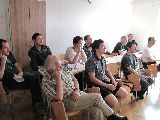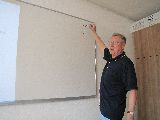Datum in ura / Date and time: 24.5.12
(13:00-13:45)
Predavatelj / Lecturer: Hiroki Koike
Naslov / Title: The isomorphism problem for cyclic configurations
Vsebina / Abstract:

Abstract: An incidence geometry consists on a set of points X of points and a set of blocks, or lines (subsets of X), such that two blocks intesect in at most one point. An incidence geometry is a *configuration* if every line has the same number of points and every point is incident with the same number of lines. A configuration is *cyclic* if its automorphism group contains a regular cyclic subgroup. The isomorphism problem for cyclic configurations asks for sufficient conditions to check wether two cyclic configurations are isomorphic or not. We say that two cyclic configurations are *multiplier equivalent* if some element of the multiplicative group of Z_n induces an isomorphism. A related problem is that for which n two isomorphic cyclic configurations  are multiplier equivalent. In this talk we show some known results for general cyclic combinatorial objects and we present some partial results for the latter problem.

Datum in ura / Date and time: 24.5.12
(13:45-14:30)
Predavalnica / Location: Samed Bajrić
Naslov / Title: The Representations of Vectorial Boolean Functions

Datum in ura / Date and time: 21.5.12
Predavatelj / Lecturer: dr.Ilya Ponomarenko (V. A. Steklov Institute of Mathematics,Russia)
Naslov / Title: Solvability of finite Schur groups
Vsebina / Abstract:

Let G be a Schur group, i.e. each Schur ring over G arises from
a suitable permutation group which contains a regular subgroup isomorphic
to G. Then the group G is solvable. This is a joint work with A.Vasiliev.

Datum in ura / Date and time: 17.5.12
Predavatelj / Lecturer: Alexander Vasilyev
Naslov / Title: Approach of lazy calculations in application to topological graph indices in Sage. Introduction to a new molecular class
Vsebina / Abstract:

Abstract: Previously we introduced a molecular graph class based on standard Sage graph class. The classical approach of object-oriented model proved to be not at its best on a huge amount of data. The conception of lazy calculations and some optimizations allowed us to dramatically increase the performance. We will talk about the nature of such problems in calculations and some solutions to avoid them.

Datum in ura / Date and time: 17.5.12
Predavatelj / Lecturer: Iva Antončič
Naslov / Title: Half-arc-transitive graphs of particular orders
Vsebina / Abstract:

Abstract: A graph is said to be half-arc-transitive if the automorphism group of the graph acts transitively on the vertex set and the edge set of the graph, but not on the arc set of the graph. In this talk we present results on classification of half-arc-transitive graphs of orders p, 2p, 3p, 4p, pq, 2pq, p^2, p^3, p^4 and 2p^2, where p and q are primes, with an emphasis on tetravalent graphs.

Datum in ura / Date and time: 14.5.12
Predavatelj / Lecturer: dr. Roman Nedela (Matej Bel University, Slovakia)
Naslov / Title: 6-decompositions of snarks
Vsebina / Abstract:

A snark is a cubic graph with no proper $3$-edge-colouring. In 1996, Nedela and \v Skoviera proved the following theorem:

Let $G$ be a snark with an $k$-edge-cut, $k\geq 2$, whose removal leaves two
$3$-edge-colourable components $M$ and $N$. Then both $M$ and $N$ can be completed to two snarks $\tilde M$ and $\tilde N$ of order not exceeding that of $G$ by adding at most $\kappa(k)$ vertices, where the number $\kappa(k)$ only depends on $k$. The known values of the function $\kappa(k)$ are $\kappa(2)=0$, $\kappa(3)=1$, $\kappa(4)=2$ (Goldberg, 1981), and $\kappa(5)=5$ (Cameron, Chetwynd, Watkins, 1987). The value $\kappa(6)$ is not known and is apparently difficult to calculate. In 1979, Jaeger conjectured that there are no 7-cyclically-connected snarks. If this conjecture holds true, then $\kappa(6)$ is the last important value to determine. Our talk is aimed attacking the problem of determining $\kappa(6)$ by investigating the structure and colour properties of potential complements in $6$-decompositions of snarks. We find a set of $14$ complements that suffice to perform $6$-decompositions of snarks with at most $30$ vertices. We show that if this set is not complete to perform $6$-decompositions of all  snarks, then $\kappa(6)\geq 20$ and there are strong restrictions on the structure of (possibly) missing complements.
Part of the proofs are computer assisted.10.05.2012 Lecturer: Nina Chiarelli

Title: Split graphs and threshold graphs
Abstract: The chromatic number of a graph is the minimum number of colors needed to color the vertices of the graph in such a way that no adjacent vertices have the same color. A clique in a graph is a subset of pairwise adjacent vertices. Graphs for which the chromatic number
of every induced subgraph is equal to the size of its largest clique, are called perfect graphs. In this talk we will give basic definitions and present different characterizations (with forbidden induced subgraphs and with degree sequences) for split graphs and threshold graphs, two of the many families of perfect graphs.

Title: Half-arc-transitive graphs with small number of alternets
Abstract: A graph is said to be half-arc-transitive if the automorphism group of the graph acts transitively on the vertex set and the edge set of the graph, and not transitively on the set of all arcs of the graph. In this talk we will describe the notion of the alternets in the half-arc-transitive graphs, and give some results in the case when the number of alternets is small.

V ponedeljek, 7. maja 2012. ob 11:00 , bo v seminarski sobi v Galebu , v okviru raziskovalnega matematičnega seminarja, predaval prof. dr. Mihael Perman.

Vljudno vabljeni k udeležbi na predavanju!

Title: Testing the roulette wheel

Abstract: The roulette wheel in principle generates random numbers uniformly distributed on the set {0,1,2,...,36}. Mechanical imperfections or wilful manipulation can lead to deviations from uniformity in various ways. Gambling houses are interested in statistics that would detect such deviations as soon as possible with the smallest probability of false alarms. The talk will present the design and the logic behind various test statistics and some of the theoretical background. The questions lead to nice illustrations of statistical concepts like sufficiency and sequential methods. At the end we will also present an optimal strategy to take advantage of a biased wheel.

23.4.2012 Lecturer: dr. Barbara Boldin

Title: Modelling viral evolution in a heterogeneous within-host environment: insights into the HIV co-receptor switch

Abstract: From the point of view of a pathogen, a host is a structured and a heterogeneous environment. In the case of HIV, for example, the existence of spatial structure is supported by the fact that the virus is found in different tissues while environmental heterogeneity originates from the ability of the pathogen to exploit different types of immune cells. To understand how heterogeneity of the within-host environment affects viral evolution we formulate a mathematical model and investigate the conditions under which viral diversification occurs within a host. Applying the model to the case of HIV, we show that the model captures three main properties of the so called co-receptor switch that is observed in many HIV infections: the initial dominance of virus strains that infect CCR5+ cells, the late switch in some (but not all) HIV infections and the associated drop in the number of uninfected T-cells. This suggests that the co-receptor switch may be a result of gradual adaptation of the virus population to target cell heterogeneity. More generally, we argue that mathematical modelling and evolutionary ecology can help us better understand the course of some infections. The talk is based on joint work with Samuel Alizon.

In the period between 17.4.2012 and 24.4.2012 there was a minicourse

" Finite p-groups: the basics, generators, and automorphisms " (10 lectures) given by Prof. Gustavo A. Fernandez Alcober (University of the Basque Country, Bilbao, Spain).

Program:

1. Basic theory of finite p-groups.
2. Some special families of finite p-groups.
3. Generators of finite p-groups: the Frattini subgroup and the Burnside Basis Theorem.
4. Power-commutator presentations of finite p-groups.
5. Constructing automorphisms: von Dyck's theorem.
6. General structure of the automorphism group of a finite p-group
7. Automorphism groups of some known p-groups
8. Existence of outer automorphisms
9. Open problems about automorphism groups

16.04.2012 Lecturer: Rok Požar (IMFM Ljubljana).

Title: On the split structure  of lifted  groups

Abstract: Let p: X˜ → X be a regular covering projection of connected graphs, and let CTp  denote the group of covering transformations. We present an algorithm for testing whether a given group of automorphisms G˜ Aut(X˜ ) lifts along p as a split extension of CTp  by G in the case when CTp  is abelian. Next, we present an algorithm for testing whether G lifts as a split extension such that some complement of CTp within G˜ has an orbit which is a section over a G-invariant set of vertices of X . Both algorithms are part of a larger package (implemented in MAGMA)  for doing computations with graph covers.  The implementation allows computing with graphs having semiedges.

02.04.2012 Lecturer: dr. Martin Mačaj (Comenius University, Slovakia and UP FAMNIT).

Title: Nonorientable regular maps over linear fractional groups

Abstract: It is well known that for any given hyperbolic pair (k,m) there exist inﬁnitely many regular maps of valence k and face length m on an orientable surface, with automorphism group isomorphic to a linear fractional group. A nonorientable analogue of this result was known to be true for all pairs (k,m) as above with at least one even entry. In this talk we establish the existence of such regular maps on nonorientable surfaces for all hyperbolic pairs.
This is a joint work with Gareth A. Jones and Jozef Širan.

Ob 25. obletnici Erasmusa je slovenska nacionalna agencija CMEPIUS pripravila natečaj »Erasmus in jaz«. Nagradili bodo najboljši video posnetek, fotografijo ali zgodbo z delovnim naslovom »Izkušnja z Erasmus mobilnosti kot priložnosti za osebni in strokovni razvoj študenta«. Razglasitev rezultatov in podelitev dobrih nagrad bo potekala 7. maja v Mariboru  v okviru promocijske kampanje Mladi in mobilnost.

Več o natečaju izveste na spodnji povezavi http://www.cmepius.si/vzu/erasmus/natecaj-2012.aspx.

28.03.2012 Lecturer: dr. Gábor Gévay (University of Szeged, Hungary)

Title: Geometric (nk) configurations

Abstract: In the simplest case, a geometric (nk) configuration is a set of n points and n lines such that each of the points is incident with precisely k of the lines and each of the lines is incident with precisely k of the points. Instead of lines, the second subset may consist of planes, hyperplanes, circles, ellipses, etc. We discuss some construction principles, and review some recently discovered classes of such configurations. We also formulate an incidence conjecture concerning a spatial (1004) point-line configuration.

26.03.2012 Lecturer: dr. Marcin Anholcer (Poznan Univeristy of Economics, Poland)

Title: Group irregularity strength of graphs.

Abstract: We investigate the \textit{group irregularity strength} ($s_\mathcal{G}(G)$) of graphs, i.e. the smallest value of $s$ such that taking any Abelian group $\mathcal{G}$ of order $s$, there exists a function $f:E(G)\rightarrow \mathcal{G}$ such that the sums of edge labels in every vertex are distinct. We prove that for any connected graph $G$ of order at least $3$, $s_\mathcal{G} (G)=n$ if $n\neq 4k+2$ and $s_\mathcal{G} (G)\leq n+1$ otherwise, except the case of some infinite family of stars.

19.03.2012 Lecturer: dr. Oliver Schaudt (Universität zu Köln, Germany).

Title: The Price of Connectivity for Vertex Cover

Abstract: We study a graph parameter called the Price of Connectivity for Vertex Cover (PoC). It was recently introduced by Cardinal et al. and is defined as the ratio of the minimum size of a connected vertex cover and the minimum size of a vertex cover.We prove some structural results for the PoC. These results concern PoC-critical, PoC-perfect and PoC-near-perfect graphs.
Joint work with E. Camby, J. Cardinal and S. Fiorini.

12.03.2012 Lecturer: dr. Istvan Kovacs

Title: The isomorphism problem for rose window graphs

Abstract: For an integer n ≥ 3, and a, r ∈ {1, 2, ..., n − 1} with r ≠ n/2, the rose window
graph Rn (a, r) is the graph Γ, where V (Γ) = {Ai , Bi | i ∈ {0, 1, ..., n − 1}}, and E(Γ) consists of four types of edges as for every i ∈ {0, 1, ..., n − 1}: {Ai , Ai+1 }, {Ai , Bi }, {Ai+a , Bi } and {Bi , Bi+r},
where addition is taken modulo n. In this talk we consider the following problem:
given a rose window graph Rn (a, r), ﬁnd all pairs (b, s) ∈ {1, 2, ..., n−1}2 for which
Rn (b, s) is isomorphic to Rn (a, r). We present a solution using a permutation
group theoretical approach.
This is a joint work with E. Dobson and Š. Miklavič.

05.03.2012 Lecturer: dr. Marko Orel

Title: The mathematics in computed tomography (CT)

Abstract: In 1979, the Nobel Prize for Medicine and Physiology was awarded jointly to Allan
McLeod Cormack and Godfrey Newbold Hounsfield, the two pioneering scientistengineers
primarily responsible for the development, in the 1960s and early 1970s,
of computerized axial tomography, popularly known as the CAT or CT scan.
At the seminar I will present the mathematics involved in computed tomography.

27.02.2012 Lecturer: dr. Klavdija Kutnar

Title: Cayley snarks

Abstract: In this talk I will discuss the well-known conjecture that there are no snarks amongst Cayley graphs. I will present an innovative approach in solving this conjecture combining the theory  of Cayley maps and the existence of independent set of vertices whose complement induces a forest in arc-transitive graphs admitting a group of automorphisms acting regularly on the set of arcs with cyclic vertex stabilizer, together with some partial results obtained thus far.
This is a joint work with Ademir Hujdurovic and Dragan Marusic.

20.02.2012 Lecturer: dr. Vito Vitrih

Title: Pythagorean-hodograph curves

Abstract: Polynomial Pythagorean-hodograph curves (PH curves) have been introduced in 1990 by Farouki and Sakkalis. They are characterized by the property that their parametric speed, i.e., the derivative of the arc length with respect to the curve parameter, is a polynomial function. Polynomial PH curves form an important class of parametric polynomial curves for which the arc length can be computed exactly and their offsets (parallel curves) are rational curves. This makes them very useful in many practical applications, e.g. in CAD/CAM systems, robotics, animation, NC machining, spatial path planning based on rotation-minimizing frames, etc.

06.02.2012 Lecturer: Hiroki Koike

Title: Isomorphic Tetravalent cyclic Haar graphs

Abstract: Let S be a subset of the cyclic group Zn. The cyclic Haar graph H(Zn,S) is the bipartite graph with vertex set two copies of the cyclic group and edges {x,y} where x and y are in Zn and x-y is in S. We give necessary and sufficient conditions for the isomorphism of two connected cyclic Haar graphs of valency 4.

30.01.2012 Lecturer: Alexander Vasilyev

Title: Introduction to Sage. Examples of application to Graph theory

Abstract: Sage (previously SAGE) is mathematical software with features covering many aspects of mathematics, including algebra, combinatorics, numerical mathematics, number theory, and calculus. In this talk we present Sage and provide some examples for usage in Graph theory. Moreover, we will talk about the stucture of the math chemistry package we are working on.

23.01.2012 Lecturer: Samed Bajrić

Title: Almost Perfect Nonlinear functions

Abstract: In this talk we present some basic properties of Almost Perfect Nonlinear (APN) functions over finite field of characteristic 2 and investigate some open problems. We provide the characterizations of Almost Perfect Nonlinear functions and of APN permutations by means of their component functions, which can be used in an iterated secret-key block cipher as a round function to protect it from a differential cryptanalysis. We conclude with the list of all, up to equivalence, APN and Almost Bent (AB) functions, which are equivalent to certain power functions f: GF(2^n) --> GF(2^n), f(x) = x^k.

16.01.2012 Lecturer: dr. Gyorgy Kiss (Eötvös Loránd University, Budapest).

Title: Semiarcs and semiovals in PG(2, q)

Abstract : Ovals, k-arcs and semiovals of ﬁnite projective planes are not only interesting geometric structures, but they have important applications to coding theory and cryptography, too. Semiarcs are the natural generalizations of arcs. Let Πq be a projective plane of order q. A non-empty pointset St ⊂ Πq is called a t-semiarc if for every point P ∈ St there exist exactly t lines  1 , 2 , . . . t such that St ∩ i = {P } for i = 1, 2, . . . , t. These lines are called the tangents to St at P. The classical examples of semiarcs are the semiovals (t = 1) and the subplanes (t = q − m, where m is the order of the subplane.)      In this talk we consider those semiarcs in PG(2, q), which are contained in the union of three concurrent lines. Applying theorems coming from additive group theory, we prove bounds on the sizes of these semiarcs and construct new examples. We investigate strong semiovals, too. For p prime, the complete classiﬁcation of strong semiovals in PG(2, p2 ), and the proof of the nonexistence of these objects in PG(2, p) was given in [?]. We extend these results for arbitrary powers of p. In particular we prove that there is no strong semioval in PG(2, p2r+1 ) if p > 7, and prove that the size of each strong semioval in PG(2, p2r ) is 3(p2r − pr ).

09.01.2012 Lecturer: dr. Gabriel Verret

Title: Constructing a census of small cubic vertex-transitive graphs.

Abstract: We describe some recent theoretical results and various tricks which allowed us to compute a census of all cubic vertex-transitive graphs of order at most 1280. We also discuss how these methods could be expanded to higher order or valency. This is joint work with Primoz Potocnik and Pablo Spiga.